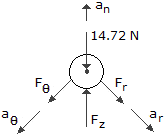# Engineering Mechanics - KOP: Force and Acceleration - Discussion

### Discussion :: KOP: Force and Acceleration - General Questions (Q.No.16)

16.A particle having a mass of 1.5 kg, moves along a three-dimensional path defined by the equations r = 94 + 3t) m,= (t2 + 2) rad, and z = (6 - t3) m, where t is in seconds, and the z-axis is vertical. Determine the r,, and z components of force which the path exerts on the particle when t = 2 s.

 [A]. Fr = 0, F2 = 30 N, Fz = -18.00 N [B]. Fr = -240 N, F2 = 66.0 N, Fz = -3.29 N [C]. Fr = 0, F2 = 3 N, Fz = -18.00 N [D]. Fr = -160.0 N, F2 = 44.0 N, Fz = -12.00 N

Explanation:

No answer description available for this question.